# 三稳态能量收集系统的同宿分岔及混沌动力学分析*

(1. 中北大学 理学院 力学系， 太原 030051；2. 上海大学 上海市应用数学和力学研究所， 上海 200072；3. 西北工业大学 工程力学系， 西安 710072)

## 1 模 型 分 析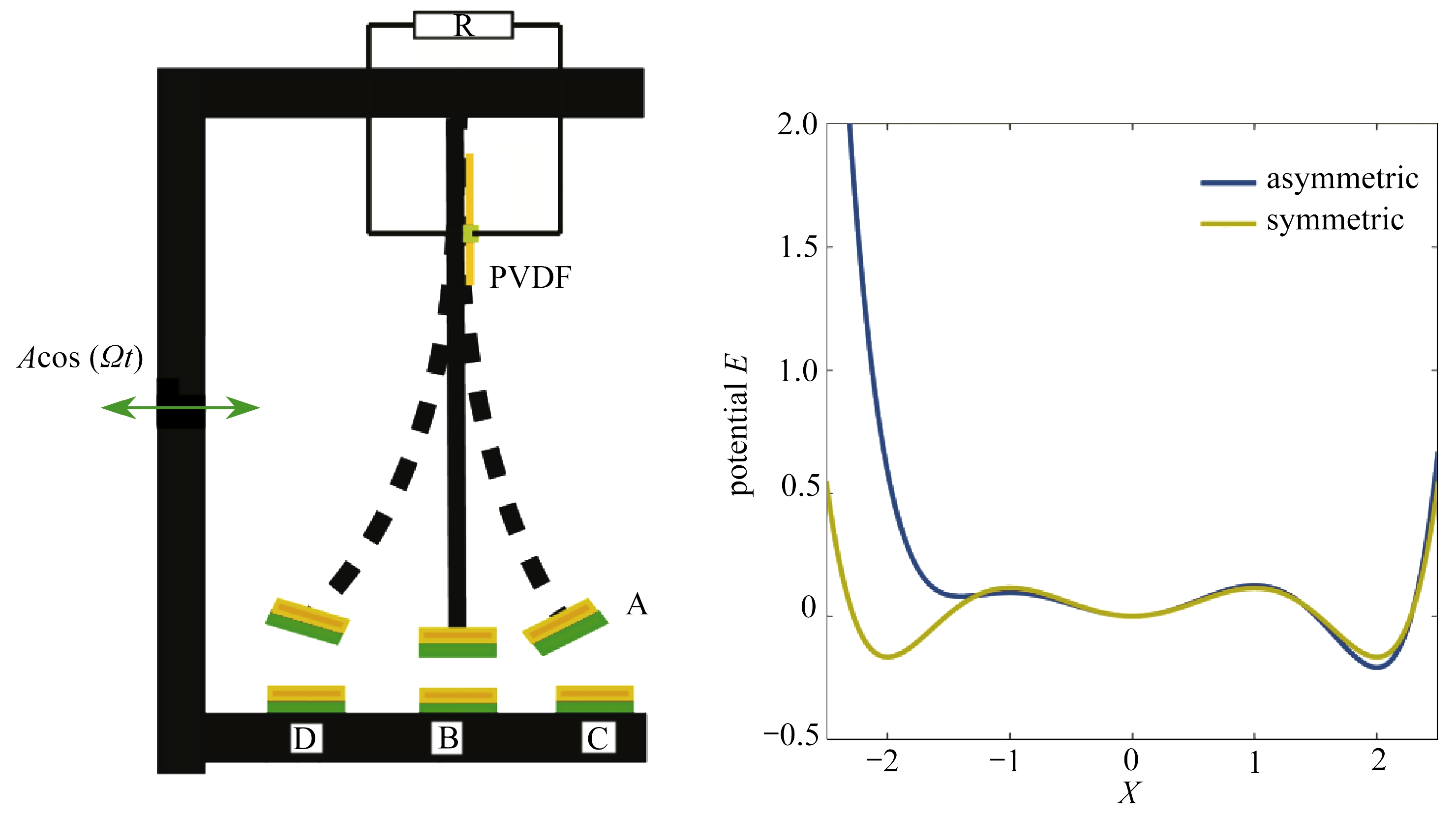(a) 模型示意图 (b) 势能函数图
(a) Schematic diagram of the model (b) The potential function diagram

Fig. 1 The schematic diagram and potential function diagram of the tristable energy harvester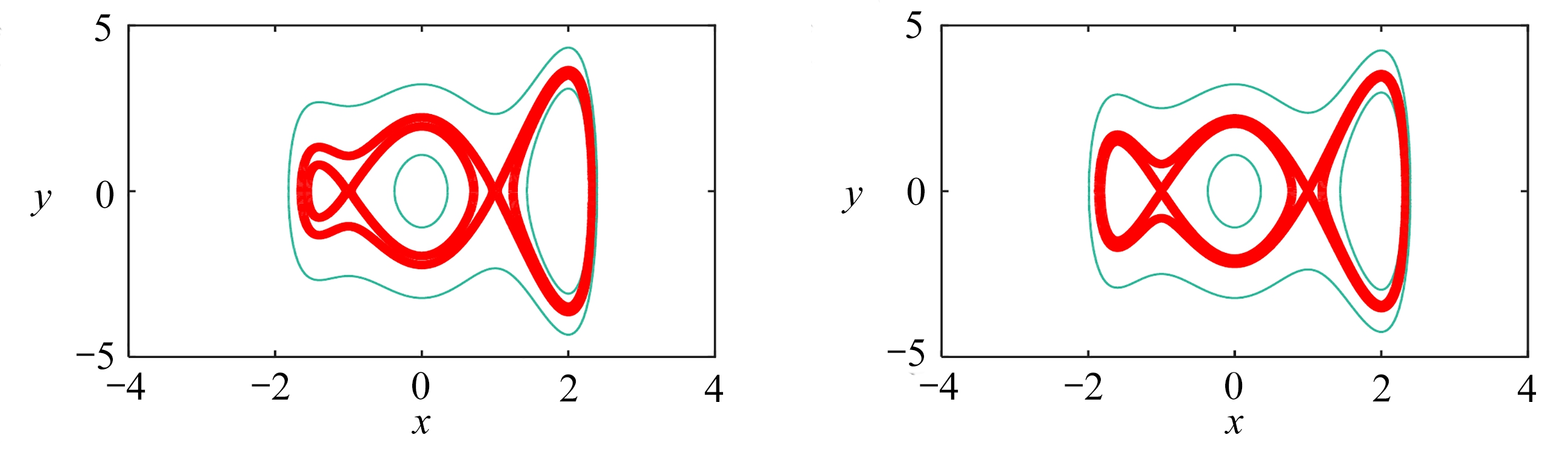(a) α3=-1.4 (b) α3=-1.6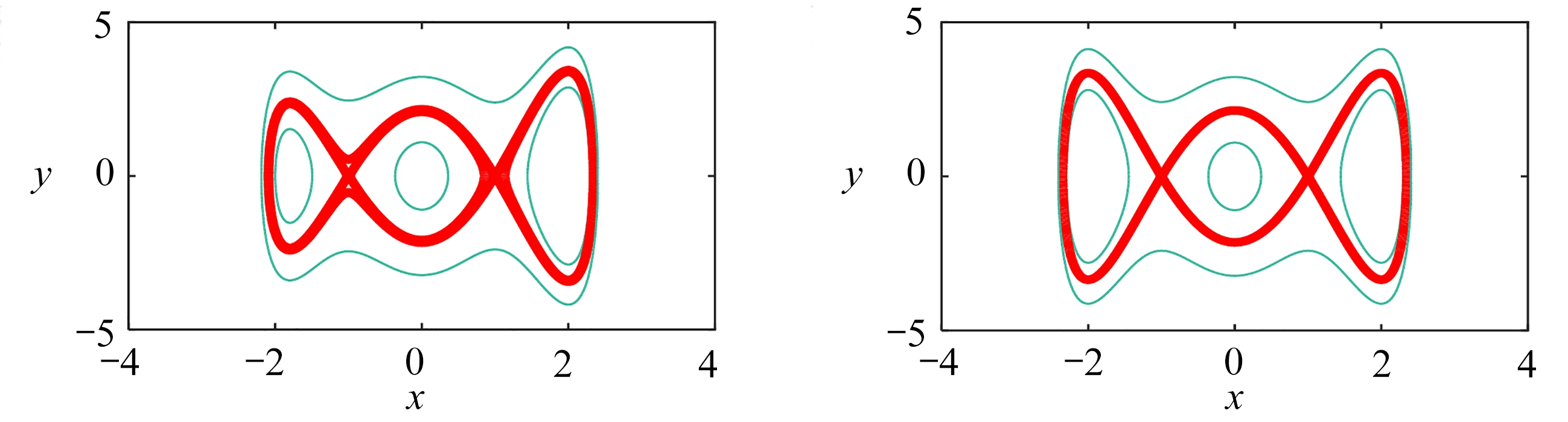(c) α3=-1.8 (d) α3=-2

Fig. 2 Phase portraits of the undisturbed Hamiltonian system for α1=-1，α2=2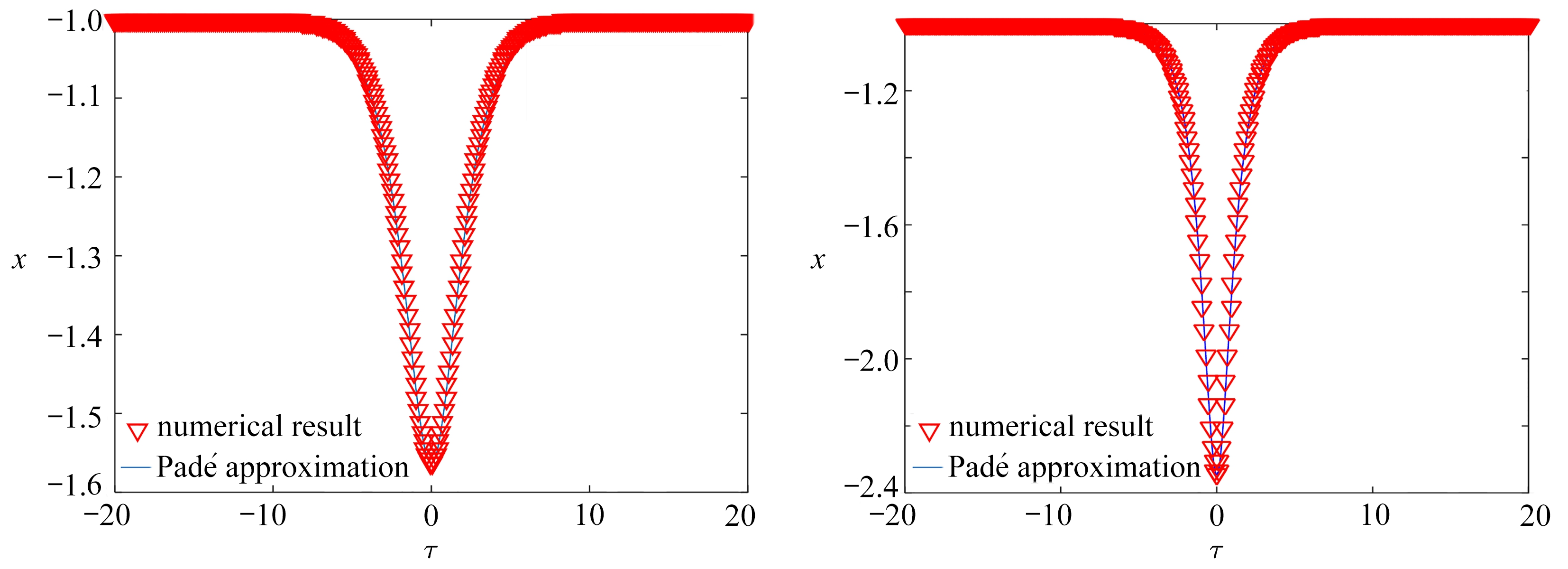(a) α3=-1.4时的同宿解 (b) α3=-2时的同宿解
(a) Homoclinic solutions for α3=-1.4 (b) Homoclinic solutions for α3=-2

Fig. 3 The comparison between approximation results and numerical results of the homoclinic orbit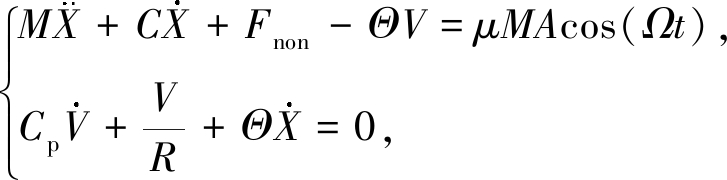(1)

Fnon=K1X+K2X2+K3X3+K4X4+K5X5

(2)

Fnon=K5(X-x1)(X-x2)(X-x3)(X-x4)(X-x5),

(3)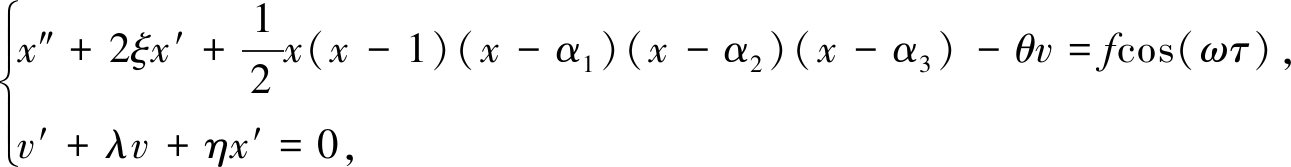(4)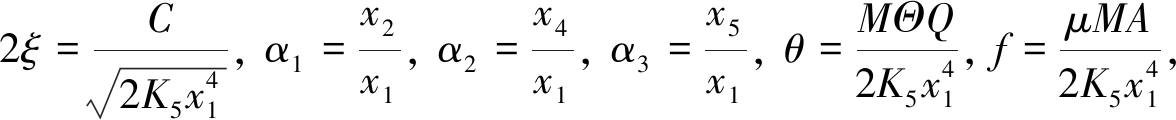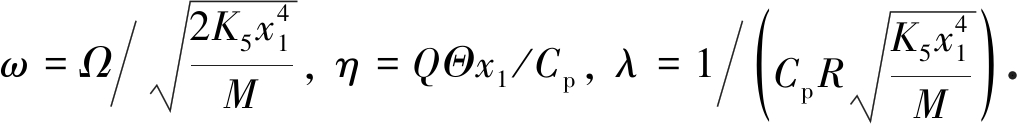x′=y，方程(4)重新写成状态方程形式: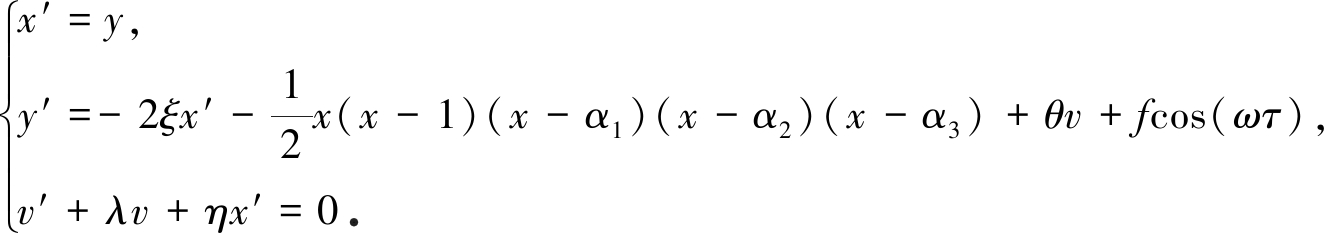(5)

## 2 Melnikov方法分析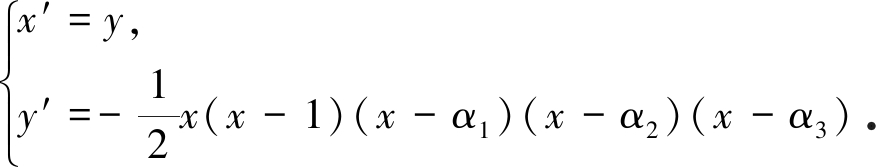(6)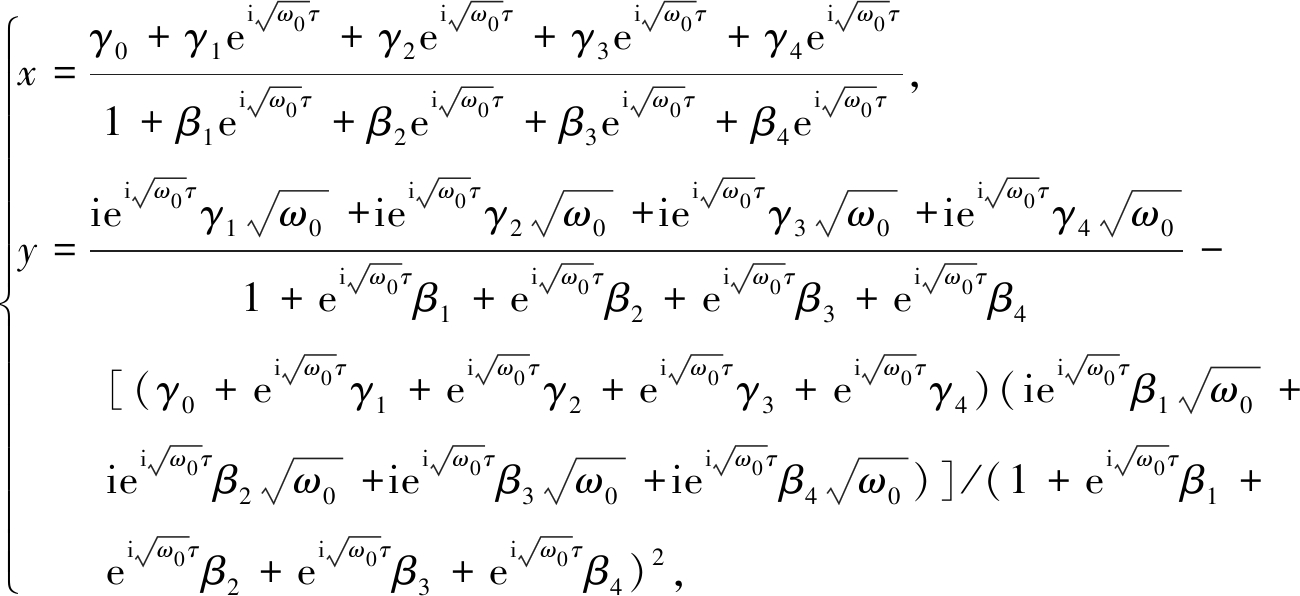(7)

Table 1 Coefficients of the Padé approximation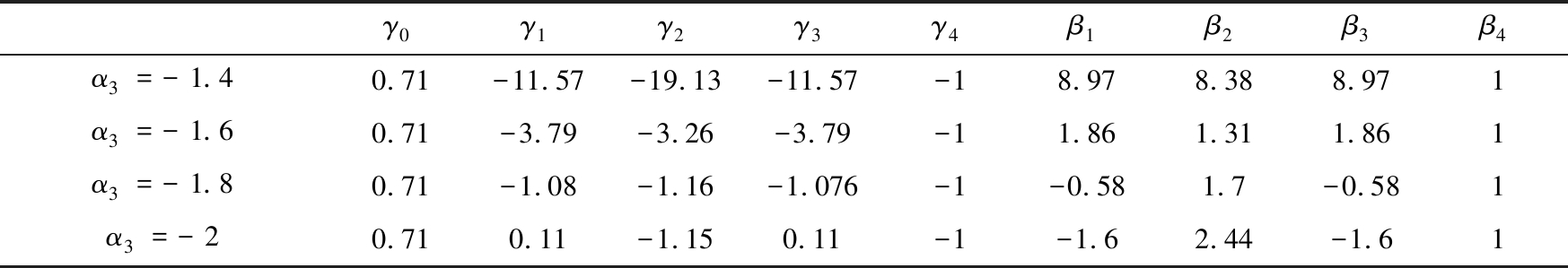γ0γ1γ2γ3γ4β1β2β3β4α3=-1.40.71-11.57-19.13-11.57-18.978.388.971α3=-1.60.71-3.79-3.26-3.79-11.861.311.861α3=-1.80.71-1.08-1.16-1.076-1-0.581.7-0.581α3=-20.710.11-1.150.11-1-1.62.44-1.61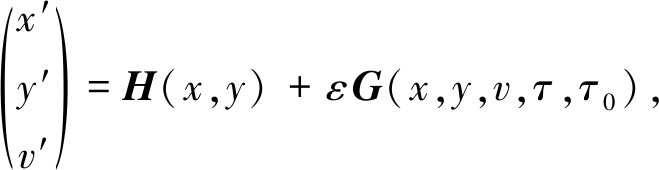(8)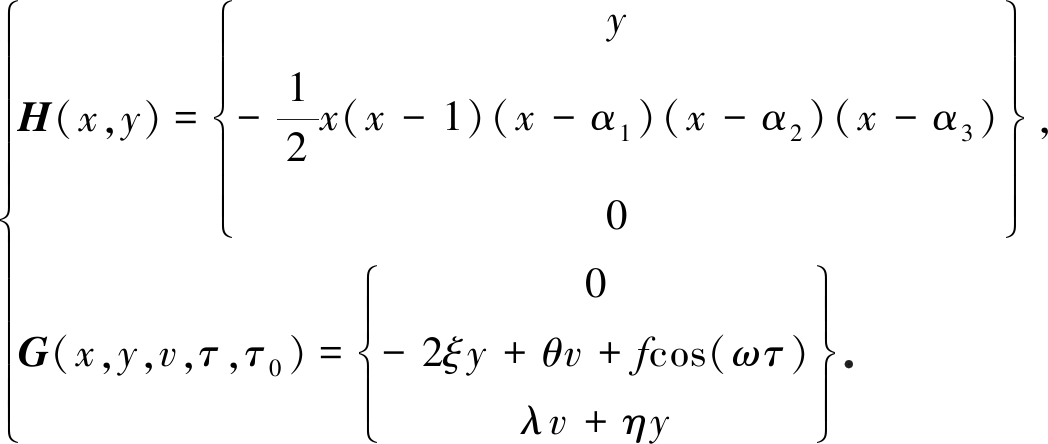(9)

M(τ0)=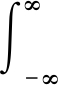H(x,y)∧G(x,y,v,τ,τ0)dτ=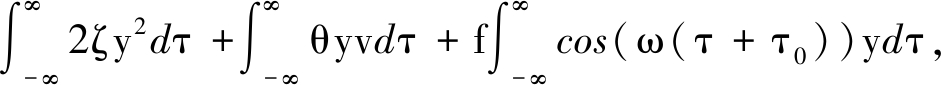(10)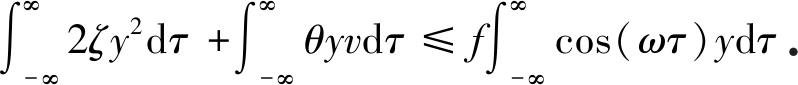(11)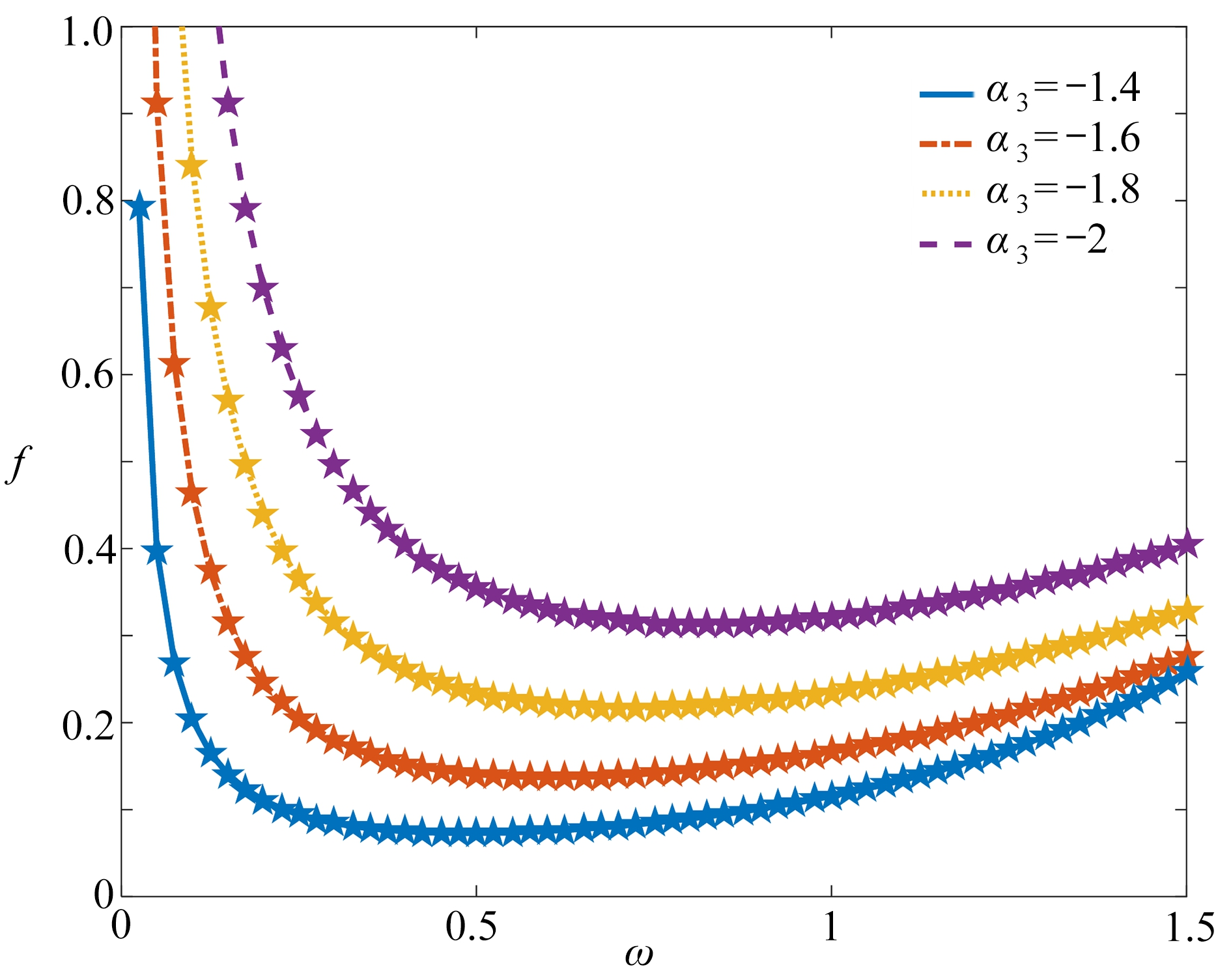Fig. 4 The homoclinic bifurcation threshold curves

## 3 数 值 模 拟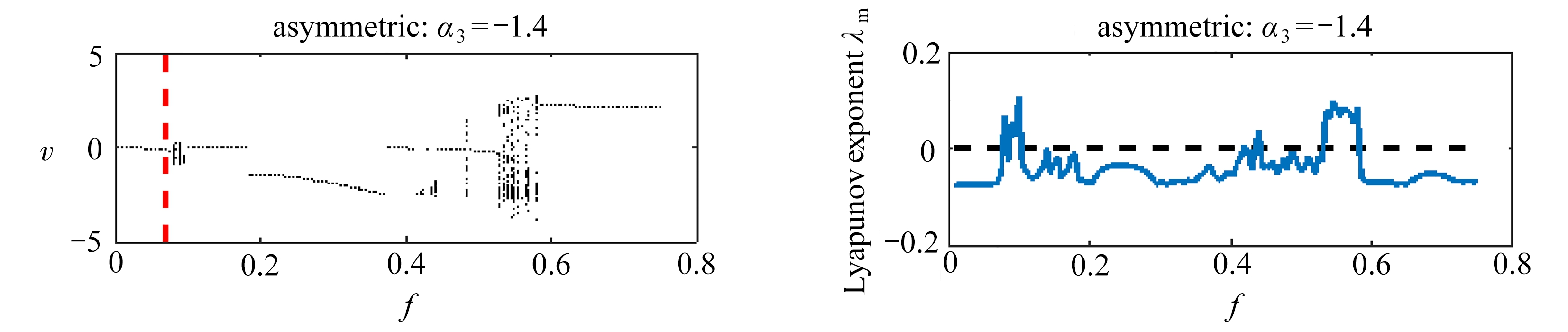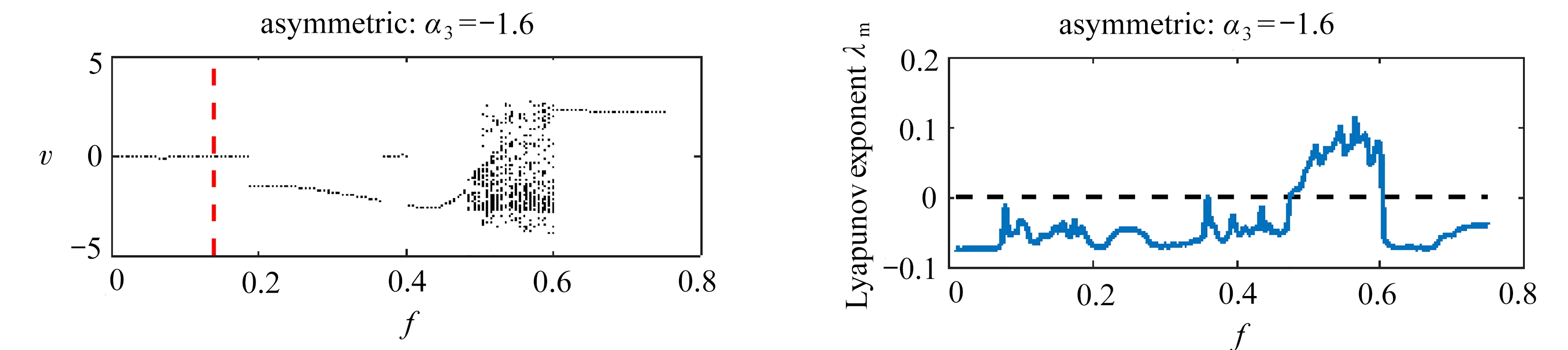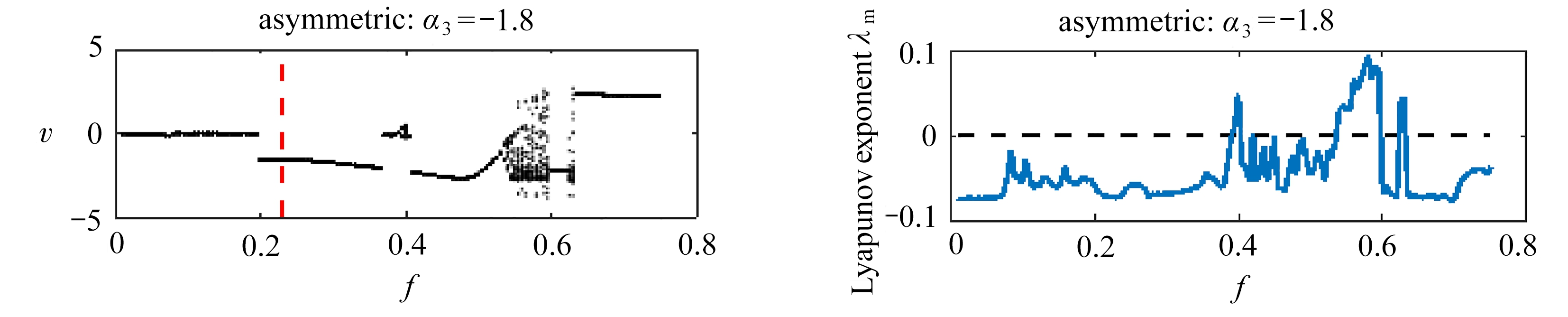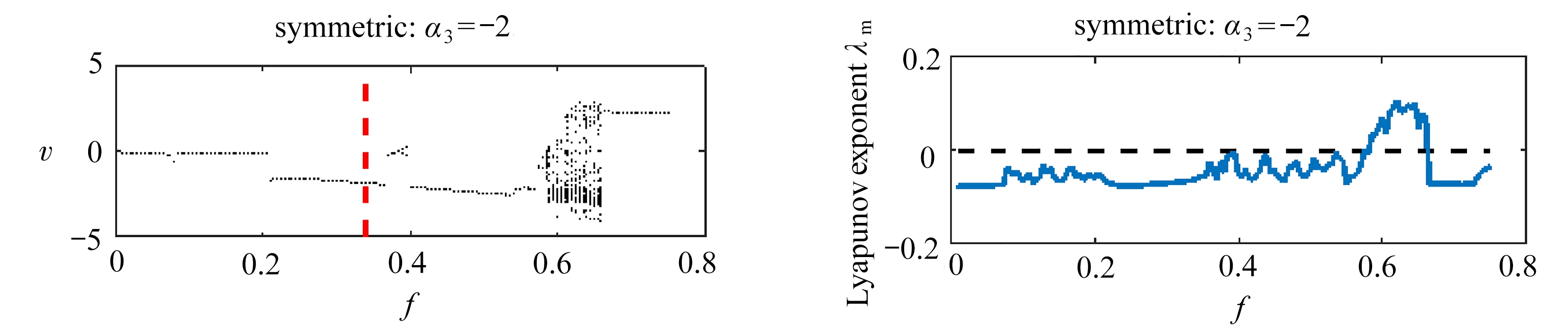(a) 分岔图 (b) 最大Lyapunov指数
(a) Bifurcation diagrams vs. f (b) Maximum Lyapunov exponents vs. f

Fig. 5 Numerical verification of homoclinic bifurcation thresholds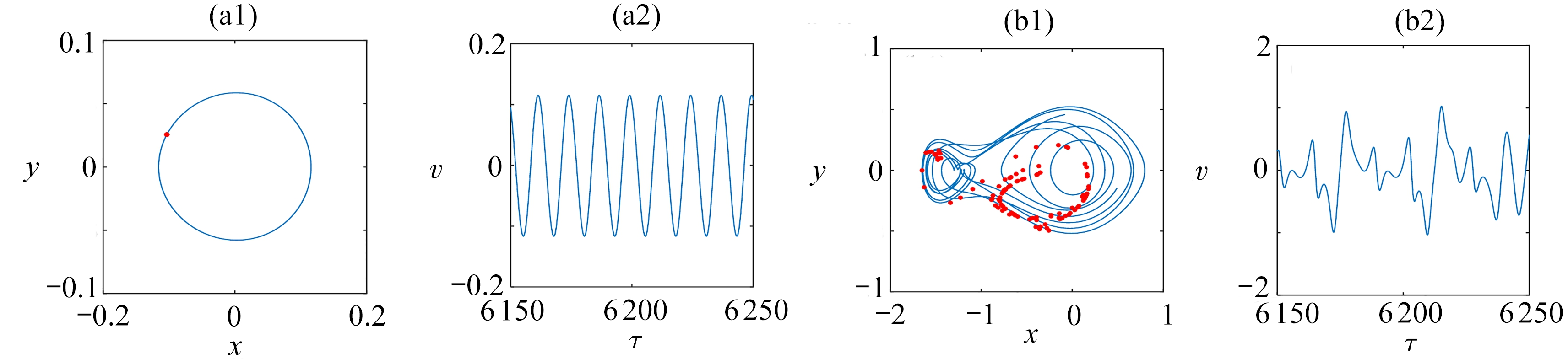(a) f=0.05,非对称情形(α3=-1.4) (b) f=0.09,非对称情形(α3=-1.4)
(a) The asymmetric case for α3=-1.4, f=0.05 (b) The asymmetric case for α3=-1.4, f=0.09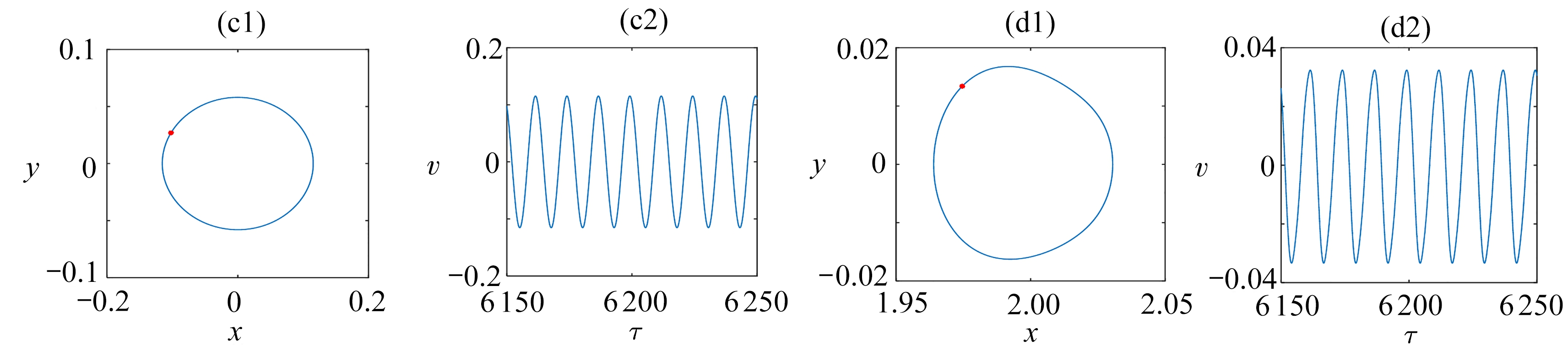(c) f=0.05,对称情形(α3=-2) (d) f=0.09,对称情形(α3=-2)
(c) The symmetric case for α3=-2, f=0.05 (d) The symmetric case for α3=-2, f=0.09

Fig. 6 The time histories and phase portraits with Poincaré sections for f=0.05 and f=0.09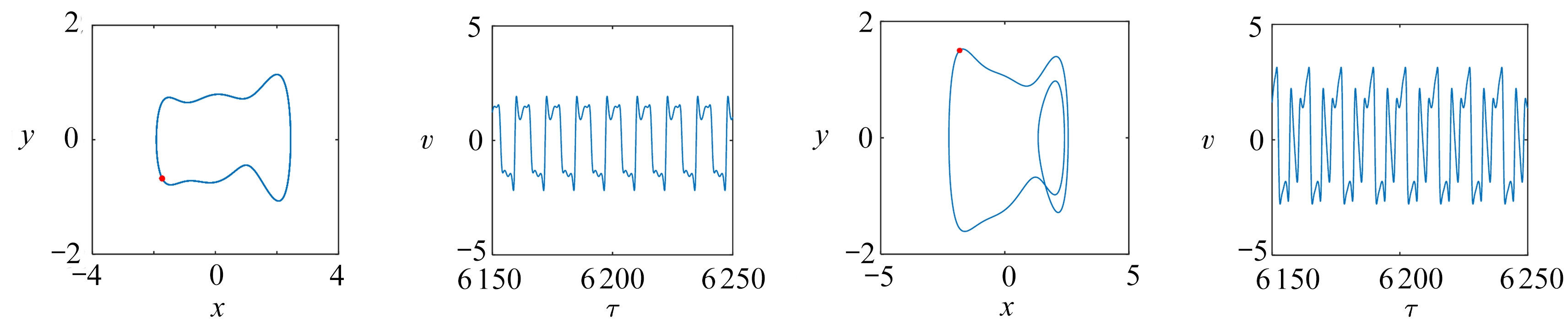(a) f=0.2,非对称情形(α3=-1.4) (b) f=0.61,非对称情形(α3=-1.4)
(a) The asymmetric case for α3=-1.4, f=0.2 (b) The asymmetric case for α3=-1.4, f=0.61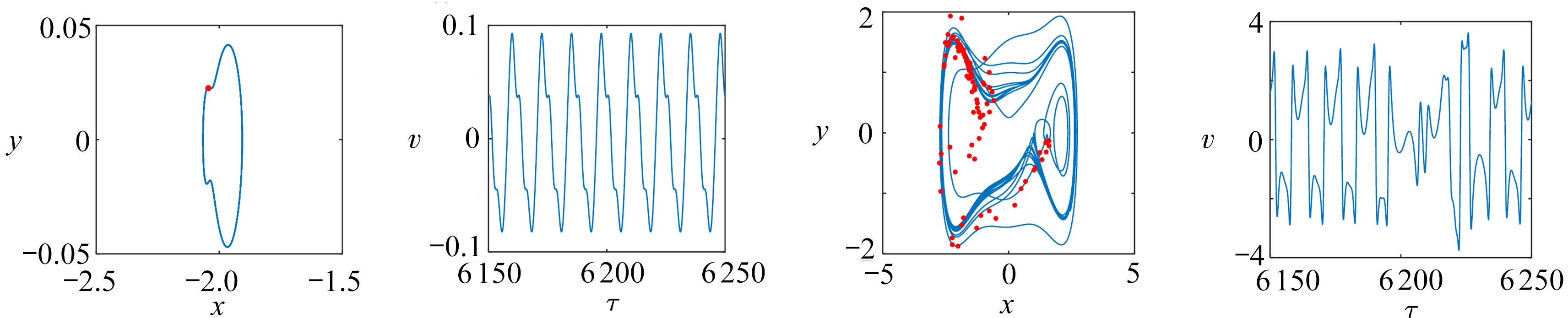(c) f=0.2,对称情形(α3=-2) (d) f=0.61,对称情形(α3=-2)
(c) The symmetric case for α3=-2, f=0.2(d) The symmetric case for α3=-2, f=0.61

Fig. 7 The time histories and phase portraits with Poincaré sections for f=0.2 and f=0.61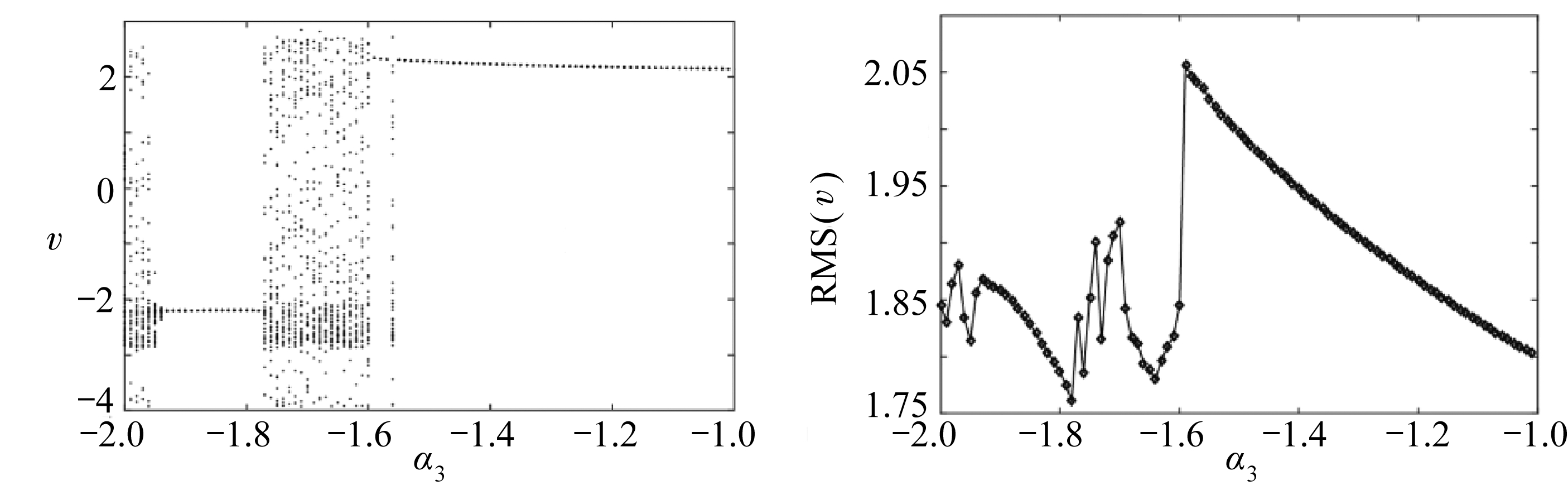(a) 随参数α3变化的分岔图 (b) 随参数α3变化的有效电压
(a) The diagram of bifurcation vs. α3 (b) The effective voltage vs. α3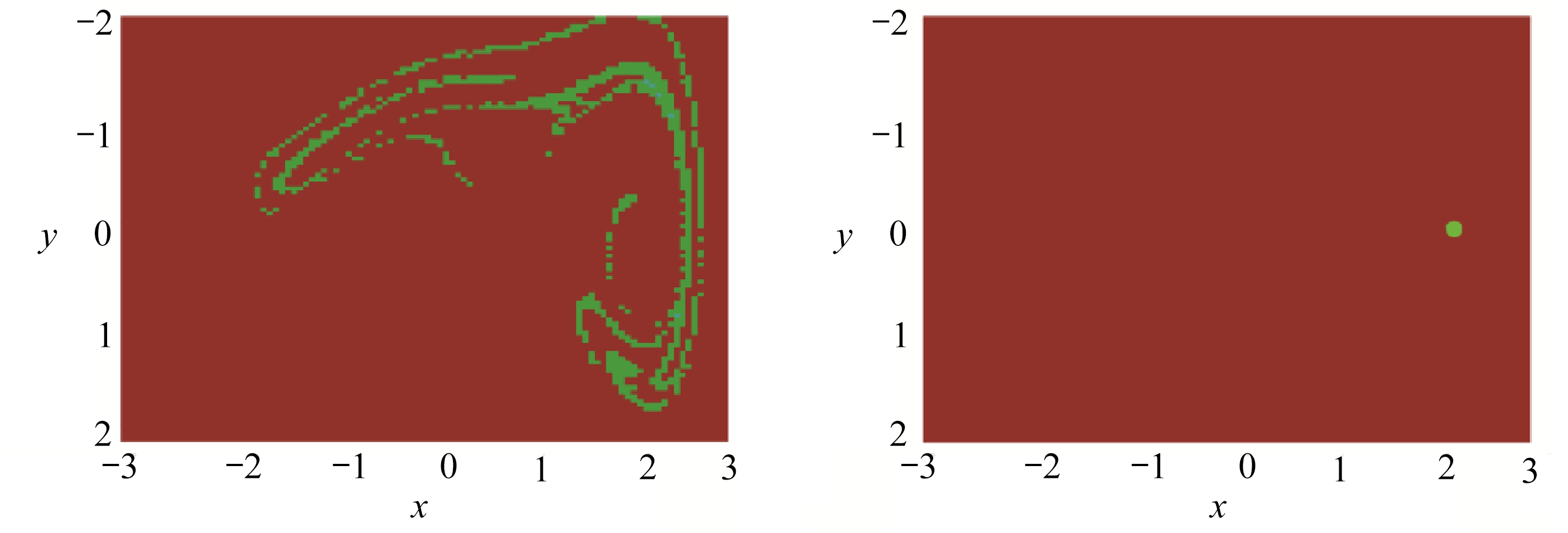(c) α3=-1.75时的吸引盆 (d) α3=-1.3时的吸引盆
(c) The basin for α3=-1.75 (d) The basin for α3=-1.3

Fig. 8 The dynamic responses influenced by parameter α3

## 4 结 论

1) 关于三稳态能量收集系统同宿分岔的理论分析与分岔图、最大Lyapunov指数等数值结果相一致，当激励强度超过同宿分岔阈值后，系统出现输出能量较高的大幅阱间振动响应．

2) 非对称势能函数能降低发生同宿分岔的阈值，提高低强度激励下的能量输出．在较低的激励强度作用下，带有非对称势能函数的三稳态能量收集系统能产生阱间的混沌以及周期响应，而对称系统的响应限制在单个的势能阱．

3) 非对称势能函数在一定程度上有助于调控三稳态能量收集系统的响应特性， 当激励强度较大时， 非对称势能函数也可将混沌运动调整为高能的大幅周期运动， 提高收集到的能量品质．

 PRIYA S, INMAN D J. Energy Harvesting Technologies[M]. New York: Springer, 2009.

 董彦辰, 张业伟, 陈立群. 惯容器非线性减振与能量采集一体化模型动力学分析[J]. 应用数学和力学, 2019, 40(9): 968-979.(DONG Yanchen, ZHANG Yewei, CHEN Liqun. Dynamic analysis of the nonlinear vibration absorber-energy harvester integration model with inerter[J]. Applied Mathematics and Mechanics, 2019, 40(9): 968-979.(in Chinese))

 HARNE R L, WANG K W. A review of the recent research on vibration energy harvesting via bistable systems[J]. Smart Materials and Structures, 2013, 22(2): 023001.

 张小静, 刘丽兰, 任博林, 等. 势阱深度对双稳态电磁发电系统发电性能的影响研究[J]. 应用数学和力学, 2017, 38(6): 622-632.(ZHANG Xiaojing, LIU Lilan, REN Bolin, et al. Influence of potential well depth on power generation performance of bistable electromagnetic energy harvesting systems[J]. Applied Mathematics and Mechanics, 2017, 38(6): 622-632.(in Chinese))

 吴子英, 牛峰琦, 刘蕊, 等. 有色噪声激励下双稳态电磁式振动能量捕获器动力学特性研究[J]. 应用数学和力学, 2017, 38(5): 570-580.(WU Ziying, NIU Fengqi, LIU Rui, et al. Dynamic characteristics of bistable electromagnetic vibration energy harvesters under colored noise excitation[J]. Applied Mathematics and Mechanics, 2017, 38(5): 570-580.(in Chinese))

 刘蕊, 吴子英, 叶文腾. 附加非线性振子的双稳态电磁式振动能量捕获器动力学特性研究[J]. 应用数学和力学, 2017, 38(4): 432-446.(LIU Rui, WU Ziying, YE Wenteng. Dynamics research of bistable electromagnetic energy harvesters with auxiliary nonlinear oscillators[J]. Applied Mathematics and Mechanics,2017, 38(4): 432-446.(in Chinese))

 MASANA R, DAQAQ M F. Energy harvesting in the super-harmonic frequency region of a twin-well oscillator[J]. Journal of Applied Physics, 2012, 111(4): 044501.

 LI H T, QIN W Y, LAN C B, et al. Dynamics and coherence resonance of tri-stable energy harvesting system[J]. Smart Materials and Structures, 2016, 25(1): 015001.

 LI H T, QIN W Y, ZU J, et al. Modeling and experimental validation of a buckled compressive-mode piezoelectric energy harvester[J]. Nonlinear Dynamics, 2018, 92(4): 1761-1780.

 ZHOU S X, CAO J Y, ERTURK A, et al. Enhanced broadband piezoelectric energy harvesting using rotatable magnets[J]. Applied Physics Letters, 2013, 102(17): 173901.

 周生喜, 曹军义, ERTURK A, 等. 压电磁耦合振动能量俘获系统的非线性模型研究[J]. 西安交通大学学报, 2014, 48(1): 106-111.(ZHOU Shengxi, CAO Junyi, ERTURK A, et al. Nonlinear model for piezoelectric energy harvester with magnetic coupling[J]. Journal of Xian Jiaotong University, 2014, 48(1): 106-111.(in Chinese)

 ZHOU S X, ZUO L. Nonlinear dynamic analysis of asymmetric tristable energy harvesters for enhanced energy harvesting[J]. Communications in Nonlinear Science and Numerical Simulation, 2018, 61: 271-284.

 CHEN L Q, LI K. Equilibriums and their stabilities of the snap-through mechanism[J]. Archive of Applied Mechanics, 2016, 86(3): 403-410.

 WANG G, ZHAO Z, LIAO W H, et al. Characteristics of a tri-stable piezoelectric vibration energy harvester by considering geometric nonlinearity and gravitation effects[J]. Mechanical Systems and Signal Processing, 2020, 138: 106571.

 李海涛, 秦卫阳. 双稳态压电能量获取系统的分岔混沌阈值[J]. 应用数学和力学, 2014, 35(6): 652-662.(LI Haitao, QIN Weiyang. Bifurcation and chaos thresholds of bistable piezoelectric vibration energy harvesting systems[J]. Applied Mathematics and Mechanics, 2014, 35(6): 652-662.(in Chinese))

 SUN S, CAO S Q. Analysis of chaos behaviors of a bistable piezoelectric cantilever power generation system by the second-order Melnikov function[J]. Acta Mechanica Sinica, 2017, 33(1): 200-207.

 KITIO KWUIMY C A, NATARAJ C, LITAK G. Melnikov’s criteria, parametric control of chaos, and stationary chaos occurrence in systems with asymmetric potential subjected to multiscale type excitation[J]. Chaos: an Interdisciplinary Journal of Nonlinear Science, 2011, 21(4): 043113.

 OUMBÉ TÉKAM G T, KITIO KWUIMY C A, WOAFO P. Analysis of tristable energy harvesting system having fractional order viscoelastic material[J]. Chaos: an Interdisciplinary Journal of Nonlinear Science, 2015, 25(1): 013112.

 TIAN R, ZHOU Y, ZHANG B, et al. Chaotic threshold for a class of impulsive differential system[J]. Nonlinear Dynamics, 2016, 83(4): 2229-2240.

 LI H T, ZU J, YANG Y F, et al. Investigation of snap-through and homoclinic bifurcation of a magnet-induced buckled energy harvester by the Melnikov method[J]. Chaos: an Interdisciplinary Journal of Nonlinear Science, 2016, 26(12): 123109.

 FENG J, ZHANG Q, WANG W. Chaos of several typical asymmetric systems[J]. Chaos, Solitons and Fractals, 2012, 45(7): 950-958.

# Homoclinic Bifurcations and Chaos Thresholds of Tristable Piezoelectric Vibration Energy Harvesting Systems

LI Haitao1,2, DING Hu2, CHEN Liqun2, QIN Weiyang3

(1. Department of Engineering Mechanics, School of Science, North University of China, Taiyuan 030051, P.R.China;2. Shanghai Institute of Applied Mathematics and Mechanics, Shanghai University, Shanghai 200072, P.R.China;3. Department of Engineering Mechanics, Northwestern Polytechnical University, Xian 710072, P.R.China)

Abstract: Nonlinear dynamic performances such as homoclinic bifurcation and chaos were investigated for tristable vibration energy harvesting systems. The analytical expression of the symmetric and asymmetric homoclinic solution was obtained through the Padé approximation, which was consistent with the numerical solution. According to the Melnikov theory, the qualitative method of studying the homoclinic bifurcation of the energy harvesting system with a triple well was developed, and the necessary condition for the occurrence of homoclinic bifurcation was obtained. Numerical simulations yielded bifurcation diagrams and maximum Lyapunov exponents that demonstrated the inter-well responses predicted with the Melnikov method. Compared with the system with symmetric potential energy, the system with asymmetric potential energy has a lower threshold of homoclinic bifurcation. For a low excitation level, the system with asymmetric potential energy witnesses inter-well chaos, while the response of the system with symmetric potential energy still keeps trapped in a single well. The change of symmetry of the system potential energy function improves the output voltage due to the increase in the probability of generating a large periodical inter-well oscillation response. The research on the homoclinic bifurcation of nonlinear energy harvesting systems with symmetric and asymmetric triple potential wells provides an effective tool for the parametric design of high-performance energy harvesters.

Key words: tristability; energy harvesting; Melnikov function; homoclinic bifurcation

(我刊编委陈立群来稿)

DOI: 10.21656/1000-0887.410164

* 收稿日期： 2020-06-08

(Contributed by CHEN Liqun, M. AMM Editorial Board)

Foundation item: The National Science Fund for Young Scholars of China(11902294)；China Postdoctoral Science Foundation(2018M640373)